Next: Dirichlet Problem in Spherical Up: Potential Theory Previous: Multipole Expansion

# Axisymmetric Charge Distributions

For the case of an axisymmetric charge distribution (i.e., a charge distribution that is independent of the azimuthal angle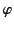), we can neglect the spherical harmonics of non-zero order (i.e., the non-axisymmetric harmonics) in Equation (335), which reduces to the following expression for the general axisymmetric Green's function: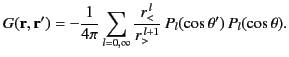(364)

Here, use have been made of the fact that [see Equation (309)]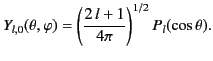(365)

In this case, the general solution to Poisson's equation, (337), reduces to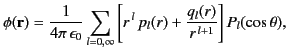(366)

where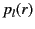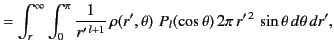(367)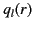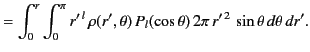(368)

Consider the potential generated by a charge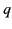distributed uniformly in a thin ring of radius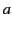that lies in the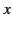-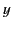plane, and is centered at the origin. It follows that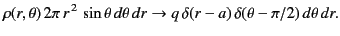(369)

Hence, for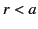we obtain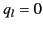and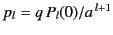. On the other hand, for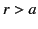we get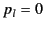and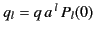. Thus,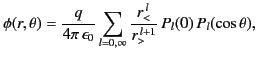(370)

where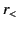represents the lesser of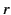and, whereas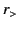represents the greater.Next: Dirichlet Problem in Spherical Up: Potential Theory Previous: Multipole Expansion
Richard Fitzpatrick 2014-06-27